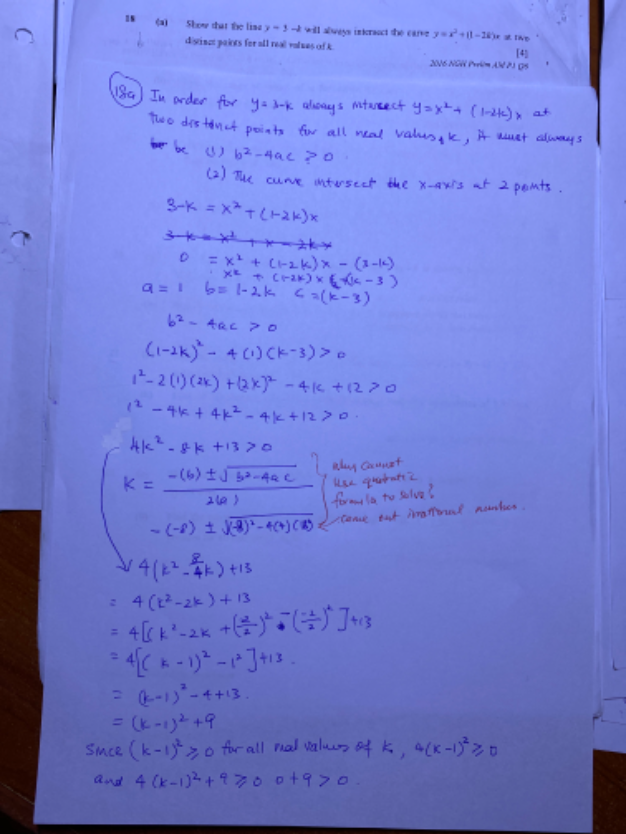# QuestionHi I have double on why we can’t use quadratic formula? And is my reasoning correct? Thanks

3-k = x2 + (1-2k) x

x2 + (1-2k) x – (3-k) =0

To find that this equation has 2 real and distinct roots, we need to prove that D > 0.

a = 1, b = (1-2k) , c = -(3-k) = k-3

(1-2k)2 – 4(1)(k-3) = 1 – 4k + 4k2 – 12 -4k

= 4k2 -8k + 13

To complete the square since it cannot be factorised,

= k– 2k + 13/4

= k– 2k  + 13/4

= k2 -2k + 1  -1 +13/4

= (k-1)2 + 9/4

So (k-1)2 and 9/4 are both positive numbers for all values of k, it implies that (k-1)2 + 9/4 > 0, hence D > 0.

Therefore,  3-k = x2 + (1-2k) x  has two real and distinct roots for all values of k.